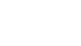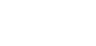# LABORATORY TESTS FOR DETERMINING STRENGTH OF ROCKSReading time: 1 minute

## Laboratory tests for determining strength of Rocks

Following are common laboratory tests for determining strength of rocks:
1. Unconfined compression tests for rocks
2. Triaxial compression test for rocks
3. Splitting tension test for rocks
4. Beam bending test for rocks
5. Ring shear test for rocks

## Unconfined Compression Test on Rocks

• It is more commonly used test for rocks to determine its strength but it should be done carefully for accurate results.
• The samples obtained during exploration are trimmed as per requirements.
• The specimen should in the cylindrical form and length to width ratio of specimen should be varying from 2 to 2.5
• The ends of specimen should be exactly perpendicular to the axis of cylinder and ends should be flat, smooth and parallel.
• The dia of specimen should not be less than 35mm. generally 45 mm dia is preferable.
• The specimen is placed in compression testing machine and subjected to compression with a stress rate of 0.5 to 1.0MPa per second.
• Note down the peak load value (P) where it fails and find out the strength of rock using below formula. Where A = initial c/s area of specimen.

q u = P/A## Triaxial Compression Test on Rocks

• This test is similar to that used for soils. Here also cylindrical specimen is required and is first subjected to lateral pressure and then deviator stress.
• As the stresses are quite large, a special type of equipment is required.
• In the usual procedure, the specimen is subjected to confining pressure (p) and then deviatoric stress is applied when P is kept constant.
• The confining pressure P increases the strength of rock. However, the increase in strength is realized only when the specimen is immersed in impervious jacket.
• Generally hydraulic oil is used as confining fluid. The jacket is made of polyurethane which is oil resistant.## Splitting Tension Test on Rocks

• This is also called as Brazilian test. In this test, the specimen is split along the diametric plane by applying load.
• The specimen having length to diameter ratio of unity when loaded on its side it splits along the diameter and parallel to the cylindrical axis. The horizontal stresses perpendicular to the loaded diameter are uniform and tensile. The tensile stress is given bywhere d = diameter, t = thickness or length of cylinder, p = applied load.

• IS: 10082-1981 recommends that the diameter of specimen should be at least 45mm and thickness of the disc shall be approximately equal to half the diameter. The load should applied at the rate of 0.2KN/sec. and the load should be measured at least to an accuracy of 1%.## Beam Bending Test on Rocks

• In this test beam is subjected to bending till failure occurs. This is also called as flexural test.
• Generally 4 –point flexural loading system is used in this test. The bottom surface of the beam is supported at two points, one near each end.
• The top portion of the beam is loaded at the third points. This system produces pure bending in the middle third of the beam.
• The flexural strength (modulus of rupture) is given bywhere P = load , L = length of specimen, d = dia of specimen## Ring Shear Test on Rocks

• This test generally used to test Insitu rocks. It gives the shear strength of rock as a function of confining pressure.
• In this test the specimen doesn’t require perfect square or smooth ends.
• Load is applied parallel to the axis of core. As the load is applied to the plunger, 2 sets of complex fractures surfaces form along the two planes of imposed shear.
• The shear strength is calculated by Tp = P/2A where p = load , A = area of cross section of specimen.